# Electric field at a point within a charged circular ring

Homework Statement:
A charged circular non-conducting ring of radius ##R## has a charge density of ##\lambda## C/m. Would the electric field at point P as shown in diagram be zero? Explain the reason for your answer.
Relevant Equations:
##E=\dfrac {kq} {r^2}## where E is electric field magnitude due to a point charge q at a point that is distant r from the charge q
I have broken the ring into a top arc and a bottom arc.

First, let's assume an imaginary charge of +1 C is placed at point P. We will determine the force on this unit charge from top and bottom arcs.

The charges in the top arc will result in electric fields that will all cancel each other perpendicular to line CP leaving us with a net electric field pointing radially inward along the line CP. This is shown in diagram.

Similarly, the charges in bottom arc will result electric field vectors that will cancel each other perpendicular to line CP leaving us with a net electric field pointing radially outward along the line CP. This is shown in diagram.

After above analysis, I am confused since it's difficult to reason whether radial outward electric field at point P will be greater than or less than or equal to the radially inward electric field at the same point.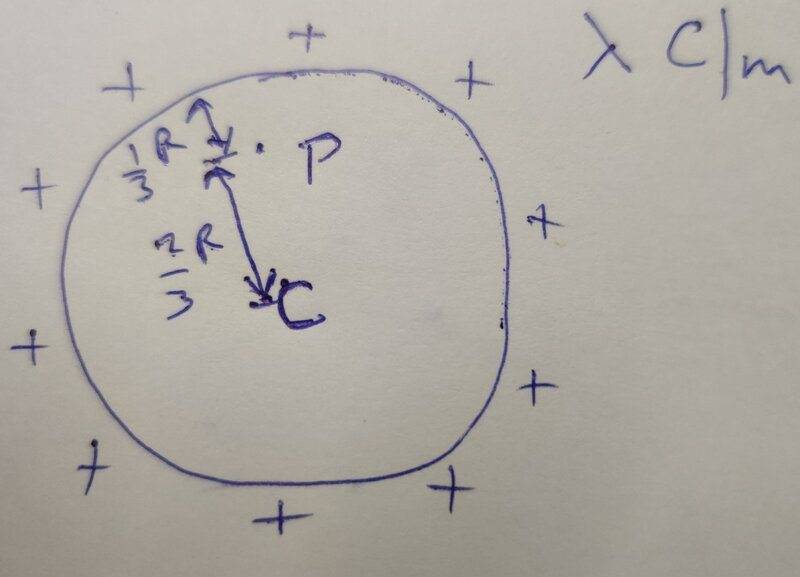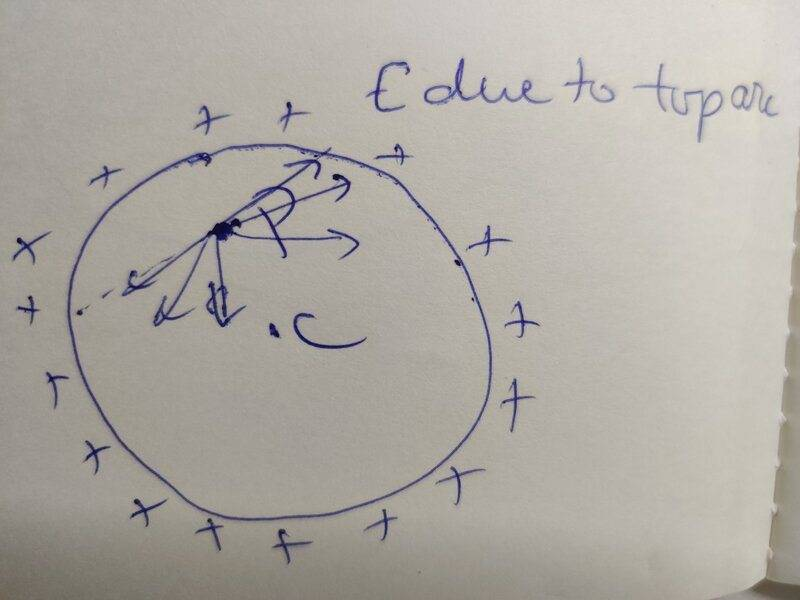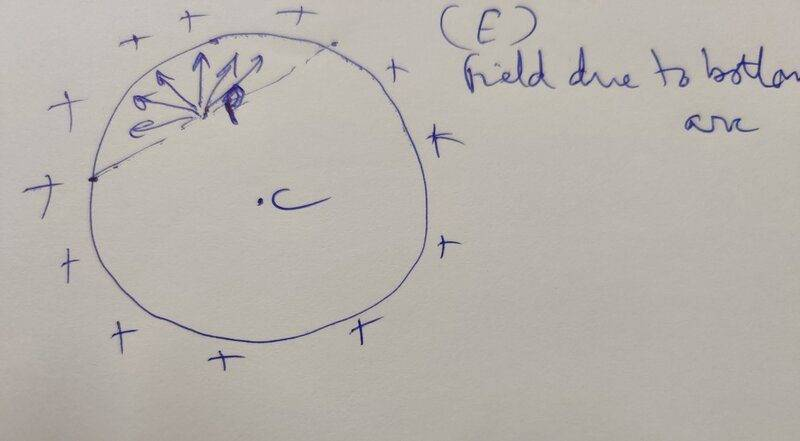Last edited:

I came up with another answer which seems like the correct one.

Consider a Gaussian surface that is a sphere centered about the center of circular ring and having a radius of ##\dfrac {2R} {3}##.

Due to Gauss's law electric flux through this Gaussian surface is zero since it has no enclosed charge.
## \oint \vec E \cdot \vec {dA} = 0##

But, how can we conclude that E = 0 at every point on the Gaussian surface from above equation of electric flux?

Last edited:
haruspex
Homework Helper
Gold Member
2020 Award
You could try writing down the integral for the net field at P and look for extrema as the distance of P from the centre of the hoop varies.

•vcsharp2003
Delta2
Homework Helper
Gold Member
I came up with another answer which seems like the correct one.

Consider a Gaussian surface that is a sphere centered about the center of circular ring and having a radius of ##\dfrac {2R} {3}##.

Due to Gauss's law electric flux through this Gaussian surface is zero since it has no enclosed charge.
## \oint \vec E \cdot \vec {dA} = 0##

But, how can we conclude that E = 0 at every point on the Gaussian surface from above equation of electric flux?
Gauss's law in integral form isn't much useful here because we can't combined it with a useful symmetry argument. There isn't spherical symmetry or cylindrical symmetry, just a circular symmetry if I can call it that way which isn't useful for Gauss's law.

I think for this problem one should write the so called "Coulomb integral" in polar coordinate system. In the plane of the ring there should be only a radial component for the electric field.

As for if the field would be radially inwards or outwards, it will be radially inwards if the ring is positively charged. To see why imagine how the electric field would look like very near the circumference of the ring and from the inside and outside of the ring.

•vcsharp2003
haruspex
Homework Helper
Gold Member
2020 Award
As for if the field would be radially inwards or outwards, it will be radially inwards if the ring is positively charged. To see why imagine how the electric field would look like very near the circumference of the ring and from the inside and outside of the ring.
It's not that simple. You could apply the same reasoning near the inside surface of a uniformly charged sphere, and we know how that turns out.

Delta2
Homework Helper
Gold Member
It's not that simple. You could apply the same reasoning near the inside surface of a uniformly charged sphere, and we know how that turns out.
Yes it is that simple. The sphere is another case, there the field is everywhere zero inside. Here it is zero only at the center.

haruspex
Homework Helper
Gold Member
2020 Award
Yes it is that simple. The sphere is another case, there the field is everywhere zero inside. Here it is zero only at the center.
Yes, we know it is zero inside the sphere, but the argument you propose in post #4 for the ring can also be applied to a sphere: think about the field inside the sphere, close to its surface and you might presume the field from the nearby charges dominates. This proves the argument is unsound.
I don't think there are any shortcuts to avoid dealing with the equations.

•SammyS
Delta2
Homework Helper
Gold Member
Yes, we know it is zero inside the sphere, but the argument you propose in post #4 for the ring can also be applied to a sphere: think about the field inside the sphere, close to its surface and you might presume the field from the nearby charges dominates. This proves the argument is unsound.
I don't think there are any shortcuts to avoid dealing with the equations.
No I don't think the argument is unsound. There must be some reason why it doesn't work in the case of the sphere. There are many differences with the sphere case, there the field at the surface of the sphere is not infinite, but in the ring it becomes infinite.

haruspex
Homework Helper
Gold Member
2020 Award
in the ring it becomes infinite.
... which you know by appealing to the equations, and was not mentioned in your argument in post #4.

Delta2
Homework Helper
Gold Member
... which you know by appealing to the equations, and was not mentioned in your argument in post #4.
Yes well, I know it because I 've done the Coulomb integral in both cases and the difference when integrating is that :
• The differential surface element is ##R^2\sin\theta d\theta d\phi## in the sphere case while the differential linear element in the ring case is ##Rd\theta##, R being the radius of the sphere or ring.
• In sphere we assume a finite surface charge density which implies an infinitesimal linear charge density (and infinitesimal charge if we consider a thin ring/circle anywhere in the sphere surface ), while in the ring we have finite linear charge density (and finite charge in the whole ring).

Last edited:
As for if the field would be radially inwards or outwards, it will be radially inwards if the ring is positively charged. To see why imagine how the electric field would look like very near the circumference of the ring and from the inside and outside of the ring.

Also, one could say that the field should be radially inward at P because electric lines of force for a +ve charge must emanate from it rather than end at it. So electric lines of force must be coming out of the +ve charges on ring as we go outwards or inwards from the ring. Does this make sense?

Gauss's law in integral form isn't much useful here because we can't combined it with a useful symmetry argument.
I get it now why Gauss's law cannot be applied. We need to have E such that a constant E can be taken out of the closed surface integral, else Gauss's law is of little use. Thankyou for clearing this symmetry concept in Gauss's law application.

•Delta2
Delta2
Homework Helper
Gold Member
Also, one could say that the field should be radially inward at P because electric lines of force for a +ve charge must emanate from it rather than end at it. So electric lines of force must be coming out of the +ve charges on ring as we go outwards or inwards from the ring. Does this make sense?
Yes this is my basic line of thinking too, coupled with the fact that the direction can not change somewhere in between along the radius from the center to the circumference because that would mean that ##\nabla\cdot\mathbf{E}\neq 0##, but we know from Gauss's law in differential form that ##\nabla\cdot\mathbf{E}=0## for all points inside and outside the ring.

•vcsharp2003
Yes well, I know it because I 've done the Coulomb integral in both cases and the difference when integrating is that :
• The differential surface element is ##R^2\sin\theta d\theta d\phi## in the sphere case while the differential linear element in the ring case is ##Rd\theta##, R being the radius of the sphere or ring.
• In sphere we assume a finite surface charge density which implies an infinitesimal linear charge density (and infinitesimal charge if we consider a thin ring/circle anywhere in the sphere surface ), while in the ring we have finite linear charge density (and finite charge in the whole ring).

Following the recommendation of applying Coulomb's law at point P, I came up with following analysis.

Consider an infinitesimal element ##dl## as shown in diagram below and determine the field ##dE## due to this element at P. We then integrate from ##\theta = 0## to ##\theta = 2 \pi## to get the net E at point P due to the charges on the ring.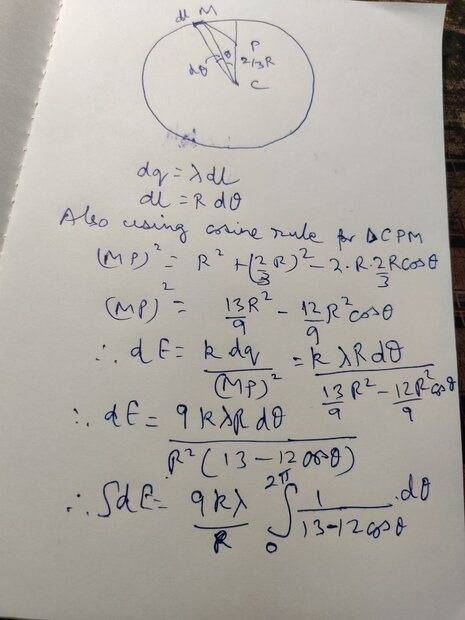Delta2
Homework Helper
Gold Member
The dE you calculate above is the magnitude of the electric field from the element ##Rd\theta##. But you need only its radial component (that is the component along the line CP). You need to multiply it by the cosine of an angle. You can find the sine of that angle by the sine law on the triangle CMP and then use ##\cos^2x+\sin^2x=1## to find the cosine.

P.S ##\sin x## will be a function of ##\sin\theta##.

•vcsharp2003
The dE you calculate above is the magnitude of the electric field from the element ##Rd\theta##. But you need only its radial component (that is the component along the line CP). You need to multiply it by the cosine of an angle. You can find the sine of that angle by the sine law on the triangle CMP and then use ##\cos^2x+\sin^2x=1## to find the cosine.

Oh, I missed that. I will look at it and rework my solution. Thankyou.

•Delta2
hutchphd
Homework Helper
There isn't spherical symmetry or cylindrical symmetry
There certainly is cylindrical symmetry!
I see nothing in the pure symmetry argument that precludes the field from being exactly zero exactly in the plane of the ring. As pointed out by @haruspex then Gauss or Maxwell must be invoked to show this impossible in the interior. But infinities are not required.

•Delta2
Delta2
Homework Helper
Gold Member
There certainly is cylindrical symmetry!
I see nothing in the pure symmetry argument that precludes the field from being exactly zero exactly in the plane of the ring. As pointed out by @haruspex then Gauss or Maxwell must be invoked to show this impossible in the interior. But infinities are not required.
There is cylindrical symmetry??? HOW? I 've done calculations and the field both in magnitude and direction clearly depends on z.
You also say that the field is zero in the plane of the ring, but my calculations don't agree with it.

hutchphd
Homework Helper
Cylindrical symmetry is invariance under rotation about z (not translation along z)

I did not say that E was zero in the plane. I said that symmetry alone does not eliminate that answer.

Delta2
Homework Helper
Gold Member
Cylindrical symmetry is invariance under rotation about z (not translation along z)

I did not say that E was zero in the plane. I said that symmetry alone does not eliminate that answer.
That's not the cylindrical symmetry I know. You seem to have some sort of weak cylindrical symmetry in mind, that is invariance only with respect to the azimuth angle ##\phi##. This weak cylindrical symmetry is not much of a use for Gauss's law in integral form.

And yes the symmetry with respect to the azimuth doesn't eliminate the case of E=0 in the interior but neither forces it.

The dE you calculate above is the magnitude of the electric field from the element ##Rd\theta##. But you need only its radial component (that is the component along the line CP). You need to multiply it by the cosine of an angle. You can find the sine of that angle by the sine law on the triangle CMP and then use ##\cos^2x+\sin^2x=1## to find the cosine.

P.S ##\sin x## will be a function of ##\sin\theta##.

I get the following that results in a complex integral.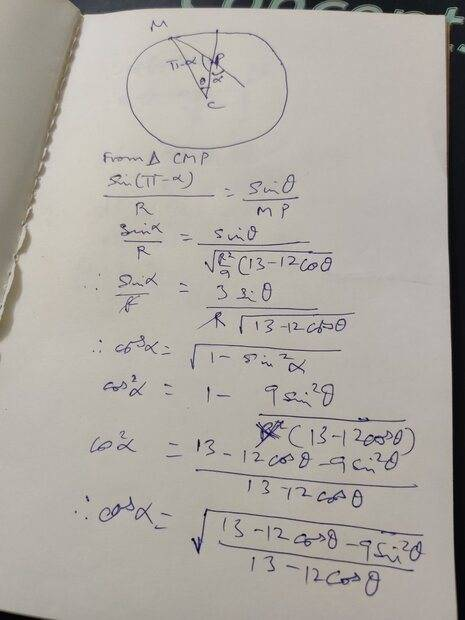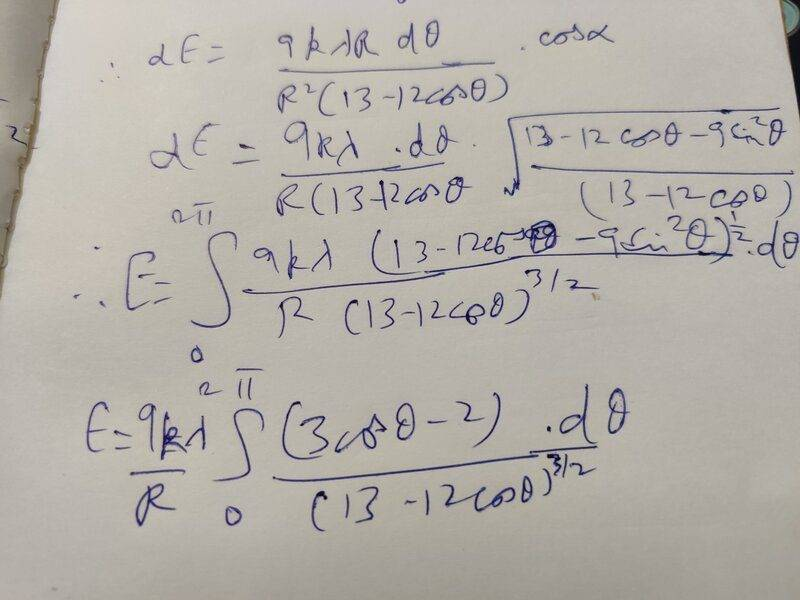Last edited:
hutchphd
Homework Helper
That's not the cylindrical symmetry I know. You seem to have some sort of weak cylindrical symmetry in mind, that is invariance only with respect to the azimuth angle ϕ. This weak cylindrical symmetry is not much of a use for Gauss's law in integral form.
I have no idea what you are talking about. What is the definition you know?

https://en.wikipedia.org/wiki/Circular_symmetry

Delta2
Homework Helper
Gold Member
I have no idea what you are talking about. What is the definition you know?

https://en.wikipedia.org/wiki/Circular_symmetry
This is one of the cases where wikipedia (and you) are wrong. The definition I know requires invariance both in ##z## and ##\phi##. Anyway I think you have to agree with me that this cylindrical symmetry you propose is of no use with Gauss's law in integral form.

•rude man
Delta2
Homework Helper
Gold Member
I get the following that results in a complex integral.

View attachment 288020

View attachment 288024
It seems correct to me what you do there except the very last step where you simplify the numerator to ##3\cos\theta-2##, what trigonometry identities you used there?

hutchphd